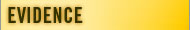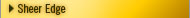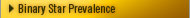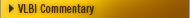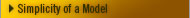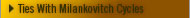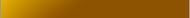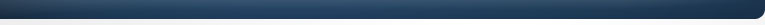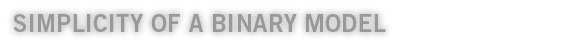Precession Model Considerations

We offer here a revised precession model based on a moving solar system, herein called the Binary Model or simply new model in this animation (you can stop or start the animations individually with the controls in the lower right hand corner). Note that both models look very similar and have similar observables.

In both models the point of equinox occurs where the celestial equator (grey disk) intersects the ecliptic (gold disk), which of course occurs twice a year - the first day of Spring and the first day of Autumn. And in both models the equinox moves at the same rate relative to the black background and fixed text (which represents the fixed stars).

The difference in the models is that the conventional model moves the point of equinox by requiring the celestial equator to slip along the ecliptic (a wobbling earth scenario as propounded by lunisolar theory), whereas in the binary model the ecliptic and celestial equator remain fixed (meaning the equinox occurs at the same point every year in the earth’s orbit path around the sun) moving against the background stars simply because the solar system moves.

The only way to easily tell the difference between the two models is by noting the position of an observer (the red X) relative to the equinox, ecliptic and celestial equator. In the conventional model the solar system is static and therefore the observer is stuck in one position (relative to the fixed stars), so the only way the equinox can move relative to the fixed stars is if the point at which the celestial equator intersects the ecliptic is constantly in local motion. In the binary model the observer remains fixed in relation to local markers and the equinox moves because the solar system moves.

The simplest observable of the precession of the equinox is that at the time of the annual equinox we observe that the sun has moved west about 50” from the position it was in the year before – relative to the background stars. Under conventional theory this motion is considered “apparent” with astronomers believing the sun did not actually move – it just “appeared” to move because the earth wobbled by 50”.

But in the new model things are much simpler. The sun moves across the sky at 50” p/y because this is how much the solar system moves per year (its angular velocity). The “precession of the equinox” then is not an “apparent” motion of the sun – it is a real motion of the sun. The solar system moves!The above graphic shows that the precession observable (seen here as the delta of the tropical and sidereal year in the lower graph) is nearly identical to the observable we find between a sidereal and tropical day (in the upper graph). It is a well known fact that the daily time difference of 4 minutes between a tropical day and a solar day is simply the result of the earth curving through space at the rate of almost one degree per day, as it orbits the sun. Likewise the annual time difference between a tropical and sidereal year can be modeled via identical orbital mechanics, albeit on a larger scale. In other words, the 20 minute difference between a tropical year and a sidereal year is simply the result of the solar system curving through space at the rate of about 50 p/y, no wobble required. The big question of course is, What does the solar system orbit?